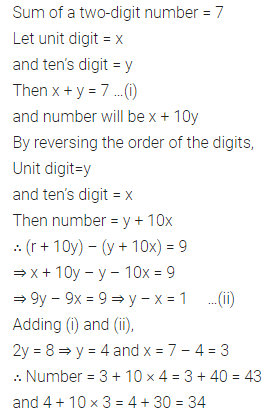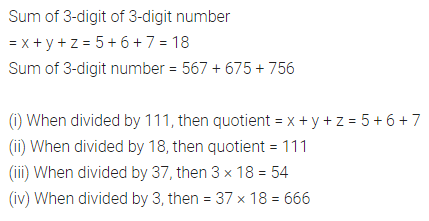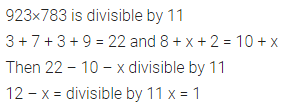# ML Aggarwal Class 8 Solutions for ICSE Maths Chapter 5 Playing with Numbers Check Your Progress

## ML Aggarwal Class 8 Solutions for ICSE Maths Chapter 5 Playing with Numbers Check Your Progress

Question 1.
In a 2-digit number, sum of digits is 7. If the difference of 2 digit number and number obtained by reversing the digits is 9, then find the number.
Solution:Question 2.
In a 3 digit number, the difference of hundred’s digit and unit’s digit is 5. Find the quotient when difference of 3-digit number and number obtained by reversing the digits is divided by 9.
Solution:Question 3.
Without actual calculation, write the quotient when sum of 3 digit numbers 567, 675 and 756 is divided by
(i) 111
(ii) 18
(iii) 37
(iv) 3
Solution:Question 4.
Find the values of the letters in each of the following and give reasons for the steps involved:Solution:Question 5.
If 923×783 is divisible by 11, what is the value of digit x?
Solution:Question 6.
Check the divisibility of following numbers by 2, 3, 9 and 11:
(i) 76543
(ii) 65432
(iii) 98765436
(iv) 234567
Solution:Question 7.
Check the divisibility of the following numbers by 5 or 10:
(i) 23565
(ii) 45270
Solution:Question 8.
Check the divisibility of the following numbers by 4 or 8:
(i) 47596
(ii) 593024
Solution: